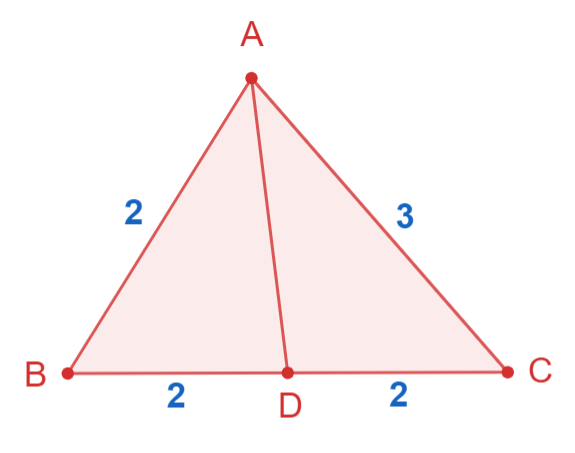Courses
Courses for Kids
Free study material
Free LIVE classes
MoreLIVE
Join Vedantu’s FREE Mastercalss

# In any triangle $AB=2, BC=4, CA=3$ and $D$ is the midpoint of $BC$, then [Roorkee 1995]A. $\cos B=\dfrac{11}{6}$B. $\cos B=\dfrac{7}{8}$C. $AD=2.4$D. $A{{D}^{2}}=2.5$Verified
90.3k+ views
Hint:
In this case, one side of the triangle has a mid-point $D$ which means that it divides the line into two equal parts. To find the correct relation for the provided data we will use the cosine rules and simplify the expression.

Formula Used:
Laws of cosine for triangle $ABC$ whose length is $a, b$, and $c$ respectively is given by;
$a^2 = b^2 + c^2 − 2bc.\cos A\\ b^2 = a^2 +c^2 − 2ac.\cos B\\ c^2 = a^2 + b^2 − 2ab.\cos C$.

Complete step-by-step solution:
We have given, triangle $AB=2, BC=4, CA=3$ and $D$ is the midpoint of $BC$ which means it divides $BC$ into two equal parts.Using the law of cosine in triangle ABD we have;
$AD^2=AB^2+BD^2-2AB.BD \cos B$
Substituting values we get;
$\cos B=\dfrac{{{2}^{2}}+{{4}^{2}}-{{3}^{2}}}{2\times 2\times 4}=\dfrac{11}{16}\\ \dfrac{11}{16}=\dfrac{{{2}^{2}}+{{2}^{2}}-A{{D}^{2}}}{2\times 2\times 2}\\ \Rightarrow A{{D}^{2}}=2.5$

Hence, $A{{D}^{2}}=2.5$.

So, option D is the correct option.

Note:
Recall that a midway divides a line into two equal segments. Students frequently mistake when attempting to determine how the given data relate to one another. It will become easier to draw the triangle with the information provided. To answer this type of question, you must be familiar with the cosine rule.

One of the most commonly applied properties in geometry is the triangle's angle sum property. Most often, the unknown angles are calculated using this attribute. The angle sum property of a triangle states that the sum of a triangle's three internal angles is $180$ degrees. A closed shape with both interior and exterior angles, a triangle is made up of three line segments. When the values of the other two angles are known, one can apply the angle sum property to determine the measure of an unknown interior angle.